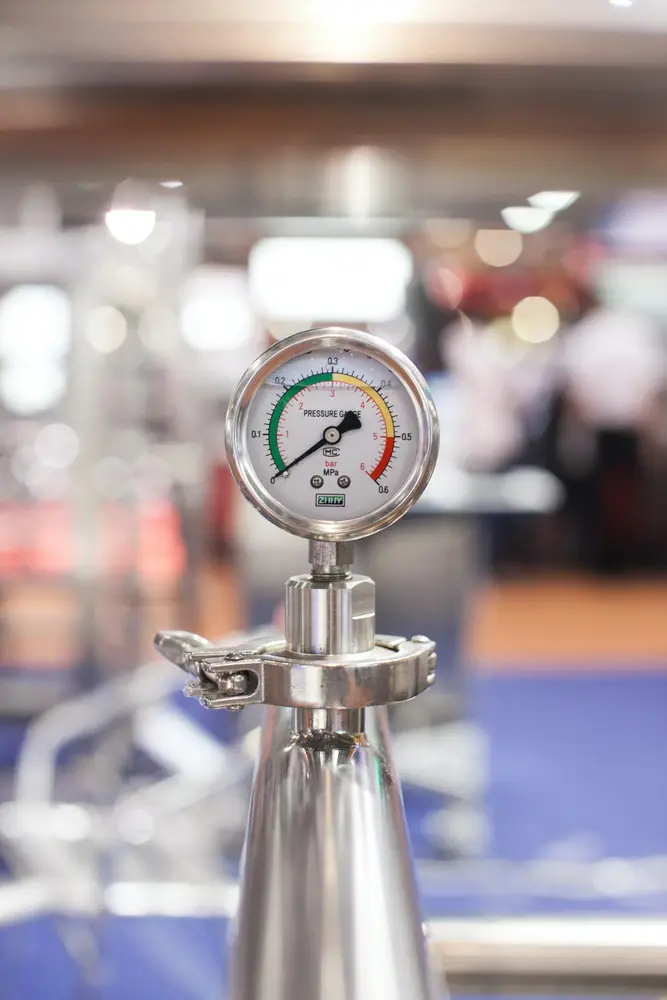# Speed and velocity

• Speed is the change of movement of an object.
• Velocity is the speed of an object in a certain direction.
• The most common unit for both speed and velocity is m/s which shows how many metres an object has moved in one second.
• Average speed = distance moved / time taken.
• Average velocity = distance moved in a certain direction / time taken.
Forces and motion

#### Acceleration

##### Acceleration

Forces and motion

#### Forces

##### Forces

Forces and motion

#### Forumulae

##### Forumulae

Forces and motion

#### Hooke's Law

##### Hooke's Law

Forces and motion

#### Momentum

##### Momentum

Forces and motion